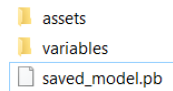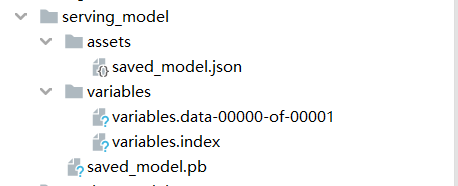• 升级到tf 2.0后, 训练的模型想转成1.x版本的.pb模型, 但之前提供的通过ckpt转pb模型的方法都不可用(因为保存的ckpt不再有.meta)文件, 尝试了好久, 终于找到了一个方法可以迂回...在tf1.x的环境下, 将tf2.0保存的weights
• 目前想要通过callback回调函数去保存pb文件？请问如何实现，能否直接保存为.pb文件，还是需要有固定的代码才可以生成pb文件？ <code>callbacks = [tf.keras.callbacks.Model...python
• 我们知道在tensorflow 2.0中...tensorflow serving 提供了很强大的部署功能，但是仅支持 .pb 格式，关于 .pb 格式的好处请参考：TensorFlow 保存模型为 PB 文件。本文说明如何用最简单的方法利用 tensorflow.keras ...
我们知道在tensorflow 2.0中利用 tensorflow.keras可以很方便地保存和加载 .h5 模型，但是 .h5格式的模型只适合在本地使用，不适合部署。tensorflow serving 提供了很强大的部署功能，但是仅支持 .pb 格式，关于 .pb 格式的好处请参考：TensorFlow 保存模型为 PB 文件。本文说明如何用最简单的方法利用 tensorflow.keras 保存为 .pd 格式的模型。
当前的博客大都采用转换的方式，转换的过程中涉及到session，但实际上，session已经被新版的tensorflow摒弃了，所以就搞得很麻烦。原来的 的tf.keras.backend.get_session() 已经变成 tf.compat.v1.keras.backend.get_session()，可见这种方法有被抛弃的趋势。
实际上，tensorflow.keras提供了一种非常简单的保存和加载 .pb 格式模型的方法。废话了这么多，上代码...
# 保存模型结构和参数到文件
tf.keras.models.save_model(network,"model_save_path") # 默认生成 .pb 格式模型，也可以通过save_format 设置 .h5 格式
print('模型已保存')
就是这么简单，保存之后会在 model_save_path 下生成如下三个文件。加载模型也非常轻松惬意:
network=tf.keras.models.load_model("model_save_path")
要将 .h5 模型转换成 .pb 格式同样非常简单，只需要先加载 .h5 再保存成 .pb 即可。
然后，就没有然后了...
展开全文keras 部署
• tensorflow2.0 保存模型方式主要有4种，下面一一介绍下。 1、保存全模型，权重和模型框架一起保存H5格式 model.save('the_save_model.h5') new_model = keras.models.load_model('the_save_model.h5') 2、将模型...
tensorflow2.0 保存模型方式主要有4种，下面一一介绍下。
1、保存全模型，权重和模型框架一起保存H5格式
model.save('the_save_model.h5')

2、将模型保存为SavedModel格式，python，java 均可调用
keras.experimental.export_saved_model(model, 'saved_model')
或者
# 保存整个模型，保存成SavedModel格式
model.save('serving_model2/', save_format='tf')
# 保存整个模型成SavedModel格式，注意参数'my_saved_model'是文件夹名称，不是文件哦！
tf.saved_model.save(model, 'serving_model/')
# 从SavedModel加载模型
print(new_model2)3、其他方式参考我上一篇博客。 【深度学习 走进tensorflow2.0】训练的模型保存方式 【深度学习 走进tensorflow2.0】训练模型以及保存最佳模型
展开全文深度学习 人工智能
• 主要介绍了tensorflow实现将ckpt转pb文件的方法，本文通过实例代码给大家介绍的非常详细，对大家的学习或工作具有一定的参考借鉴价值,需要的朋友可以参考下
• 1、保存全模型 model.save('the_save_model.h5') new_model = keras.models.load_model('the_save_model.h5') 2、保持为SavedModel文件 keras.experimental.export_saved_model(model, 'saved_model') new_...
1、保存全模型
model.save('the_save_model.h5')
new_model = keras.models.load_model('the_save_model.h5')
2、保持为SavedModel文件
keras.experimental.export_saved_model(model, 'saved_model')
new_model = keras.experimental.load_from_saved_model('saved_model')
3、仅保持网络结构，这样导出的模型并未包含训练好的参数
config = model.get_config()
reinitialized_model = keras.Model.from_config(config)
new_prediction = reinitialized_model.predict(x_test)
assert abs(np.sum(predictions-new_prediction)) >0
4、仅保存网络参数
weights = model.get_weights()
model.set_weights(weights)
# 可以把结构和参数保存结合起来
config = model.get_config()
weights = model.get_weights()
new_model = keras.Model.from_config(config) # config只能用keras.Model的这个api
new_model.set_weights(weights)
new_predictions = new_model.predict(x_test)
np.testing.assert_allclose(predictions, new_predictions, atol=1e-6)
6、保存网络权重为SavedModel格式
model.save_weights('weight_tf_savedmodel')
model.save_weights('weight_tf_savedmodel_h5', save_format='h5')

展开全文• TensorFlow2.0模型保存与调用模型保存模型调用 模型保存 首先建立一个手写数字识别的神经网络，将其训练后保存为模型文件： import tensorflow as tf from tensorflow.keras import layers # 数据处理部分，包括...


TensorFlow2.0模型保存与调用
模型保存模型调用

模型保存
首先建立一个手写数字识别的神经网络，将其训练后保存为模型文件：
import tensorflow as tf
from tensorflow.keras import layers

# 数据处理部分，包括训练集与测试集
(x_train, y_train), (x_test, y_test) = tf.keras.datasets.mnist.load_data()
x_train = x_train.reshape(60000, 28, 28, 1).astype('float32') / 255
x_test = x_test.reshape(10000, 28, 28, 1).astype('float32') / 255
y_train = tf.keras.utils.to_categorical(y_train, 10)
y_test = tf.keras.utils.to_categorical(y_test, 10)

# 通过函数式构建网络模型
encode_input = tf.keras.Input(shape=(28,28,1), name='img')
h1 = layers.Conv2D(16, 3, activation='relu', padding='SAME')(encode_input)
# 最大池化操作
h1 = layers.MaxPool2D()(h1)
# 第二层卷积
h1 = layers.Conv2D(32, 3, activation='relu', padding='SAME')(h1)
# 最大池化操作
h1 = layers.MaxPool2D()(h1)
# 将二维数据拉直成为一维数据
h1 = layers.Flatten()(h1)
# 第三层全连接操作
out = layers.Dense(10, activation='softmax')(h1)

model = tf.keras.Model(inputs=encode_input, outputs=out)

# 设置模型参数
loss='categorical_crossentropy',
metrics=['accuracy'])

# 输入数据开始训练模型
model.fit(x_train, y_train, epochs=2, batch_size=16)

# 查看模型输出结果
print(model.predict(x_test, batch_size=8))

# 保存完整模型
# .h5格式
model.save("../weights/minist.h5", save_format="")
del model

# .pb模式
#tf.saved_model.save(model, "../weights/minist")

通过model.save()函数能非常方便的保存模型，TensorFlow2.0主要包括两种方式保存完整模型：
.h5文件，具体代码model.save(“xxx.h5”)pb文件，具体代码mode.save(“xxx”, save_format=“tf”)模型调用
import numpy as np
import tensorflow as tf

(x_train, y_train), (x_test, y_test) = tf.keras.datasets.mnist.load_data()
x_train = x_train.reshape(60000, 28, 28, 1).astype('float32') / 255
x_test = x_test.reshape(10000, 28, 28, 1).astype('float32') / 255
y_train = tf.keras.utils.to_categorical(y_train, 10)
y_test = tf.keras.utils.to_categorical(y_test, 10)

# 加载pb模型

model.summary()

print("第二次输出：")
print(model.predict(x_test, batch_size=8))

对比下两次输出结果就可以知道模型是否加载正确，是不是非常简单呢！
展开全文• 零、综述 save/load weights save/load entire model saved_model 一、Save the weights 1.一次性保存所有参数 ...注意，用该方法保存模型只保存了参数，文件较小，加载较快，但是测试/部署时...
• 前言：tensorflow2.0中有很多种模型保存方法，为了做一个统一的比较，本文做一个归纳总结，加之自己在这上面踩过很多的坑，现在感觉做一个总结实在是有必要。因为tensorflow创建模型的方式很多，包括Sequence、Model...keras checkpoint
• ## TensorFlow2.0模型格式转换为.pb格式

千次阅读 多人点赞 2020-04-24 18:14:59
本文主要介绍如何将tensorflow2.0模型转化为.pb格式。
• tensorflow2.0中模型的加载更加便捷。 我在github上新建了一个有关ner的项目，其中有对tensorflow2.0的api的一些详细使用。NER 想了解更多tensorflow2.0中模型存储加载方法，可以直接到其官方网站tf2.0. 我们这里说...自然语言处理
• 最近有个具体的问题，必须把用...tf2.0训练的模型，尝试了多种方法，没有找到能用的方法去转化成pb文件； 具体原因也不明白：这里只是记录一个摸索、验证过的方法； 1. keras要用原生的，不要用 from ten...
• 主要介绍了tensorflow模型文件(ckpt)转pb文件（不知道输出节点名），本文通过实例代码给大家介绍的非常详细，对大家的学习或工作具有一定的参考借鉴价值,需要的朋友可以参考下
• 今天小编就为大家分享一篇Tensorflow读取并输出已保存模型的权重数值方式，具有很好的参考价值，希望对大家有所帮助。一起跟随小编过来看不看
• 升级到tf 2.0后, 训练的模型想转成1.x版本的.pb模型, 但之前提供的通过ckpt转pb模型的方法都不可用(因为保存的ckpt不再有.meta)文件, 尝试了好久, 终于找到了一个方法可以迂回转到1.x版本的pb模型. Note: 本方法首先...keras
• \\Users\\xiaomao\\Desktop\\1\\' + str(i) +'.jpg',show_pic) #方法2：训练模型并保存h5文件，代码来自官方链接#https://tensorflow.google.cn/guide/keras/training_keras_models_on_cloud?hl=en def train():...深度学习 神经网络 python opencv
• 文章原链接： ... 使用spark-scala调用tensorflow2.0训练好的模型 如果使用pyspark的话会比较简单，只需要在每个executor上用Python加载模型分别预测就可以了。 但工程上
• 一、保存： graph_util.convert_variables_to_constants 可以把当前session的计算图串行化成一个字节流（二进制），这个函数包含三个参数：参数1：当前活动的session，它含有各变量 参数2：GraphDef 对象，它描述了...
• 1、传统神经网络的问题 结构复杂，权值太多，计算量太大 权值太多，需要大量样本进行训练 样本的大小最好是参数数量的5~30倍 2、CNN 局部感受野 ...from tensorflow.examples.tutorials.mnist import in深度学习 图像识别
• ## YOLOv4+tensorflow2.0训练自己的数据

千次阅读 热门讨论 2021-04-01 13:44:44
YOLOv4+tensorflow2.0训练自己的数据一、环境配置1.1 实验源码1.2 环境要求二、环境测试2.1 下载权重文件2.2 权重文件转换2.3 测试三、使用自己的数据训练3.1 数据集制作3.2 在data/dataset文件夹下生成数据的描述...深度学习
• 数据预处理+VGG16迁移学习1、加载数据库2、参数设置3、读取文件中的图片4、对数据进行预处理4.1、查看数据及标签的数量5、对数据集进行划分5.1...8.1、曲线平滑处理8.2、测试集测试效果9、将模型保存pb文件三级目录...深度学习 机器学习 可视化
• 第一种方法直接用keras...import tensorflow as tf import time import datetime import json import matplotlib matplotlib.use("Agg")#这个设置可以使matplotlib保存.png图到磁盘 import matplotlib.pyplot as plt...keras 模型训练
• tensorflow2.5.0 1.1 Anaconda3-5.0.1版本安装包下载、安装流程、环境配置 参考https://www.freesion.com/article/93321279940/ 验证：点开菜单栏—>Anaconda3—>Anaconda prompt—>以管理员身份运行—&...python windows anaconda
• 保存pb文件（tf.saved_model） tf.saved_model和model.save的区别在于，tf.saved_model格式的模型可以直接用来预测，但是tf.saved_model没有保存优化器配置，而model.save保存了优化器配置，所以整体更大。 保存...
• ## 在tensorflow2.0上使用tensorrt6加速

千次阅读 热门讨论 2019-12-31 01:58:11
tensorflow2.0上训练最简单的模型并使用tensorrt加速推理引言运行环境准备工作流程例子训练和保存模型转换成tensorrt图并优化...tensorflow2.0模型可以一句话转换成savedmodel,代替之前贼麻烦的pb文件 可以通过ten...神经网络
• pb文件保存方法 使用 tf.train.Saver 会保存运行 TensorFlow 程序所需要的全部信息，然而有时并不需要某些信息。比如在测试或者离线预测时，只需要知道如何从神经网络的输入层经过前向传播计算得到输出层即可，而...
• tensorflow如何pb文件转换为h5格式保存深度学习
• 文章目录一、拉取TensorFlow Serving镜像二、模型打入TFServing镜像内部1.保存模型为pb格式2.将本地模型copy入镜像3.启动新的带有模型文件的镜像4.gRPC方式远程...首先需要将tensorflow 模型保存pb格式，通过如下代码模型部署 深度学习 docker...